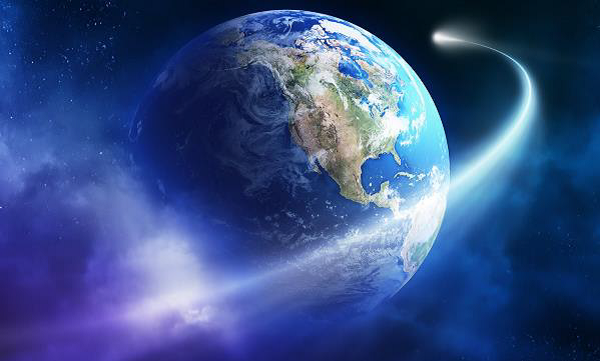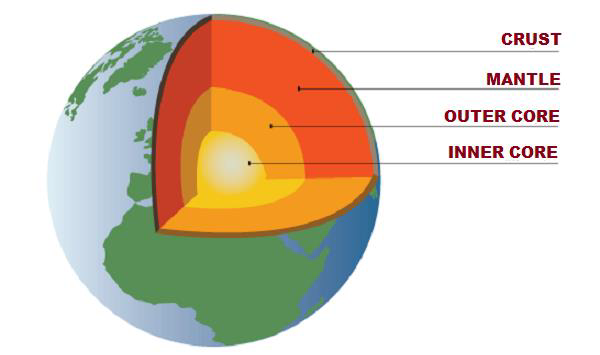# General Knowledge - Basics of Planet Earth

• Total surface area of the Earth is 510,100,500 sq. km, out of which −

• The total land area is 148,950,800 sq. km (29.08% of total area) and

• The total water area is 361,149,700 sq. km (70.92%).

• Diameter of the Earth at Equator is 12,755 km, at the poles 12,712 km, and mean diameter is 12,734 km.• Circumference of the Earth at Equator is 40,075 km and at the poles 40,024 km.

• Equatorial radius of the Earth is 6,377 km.

• The total mass of the Earth is 5.98 x 1024 kg.

• Approximate age of the Earth is 4,500 million years.

• The mean velocity of the Earth in its orbit (around the Sun) is 107,218 km/h.

• The most abundant elements of the Earth are

• Oxygen (29.8%),

• Silicon (15.6%), and

• Magnesium (13.9%)

## Structure of Earth

• The Earth is structured in three layers namely −

• Crust,

• Mantle, and

• Core – Further classified as outer core (fluid layer) and inner core (solid layer).• The Crust is the uppermost layer of the Earth, largely composed of rocks. Its thickness ranges from 5 km to 60 km and density ranges from 2.7 to 3.

• The Crust shares about 1 percent of Earth’s volume.

• The Crust is categorized as ‘Continental Crust’ and ‘Oceanic Crust.’

• The thickness of Continental Crust ranges from 30 km to 50 km. It is largely composed by granites, which density (i.e. 2.7) is lesser than the Oceanic Crust.

• The thickness of Oceanic Crust ranges from 5 km to 10 km and it is composed primarily of basalt, diabase, and gabbro.

• The density of Oceanic Crust is 3.0.

• The most abundant element of Crust is Oxygen (46.6%) followed by Silicon (27.7%) and Aluminum (8.1%).

• The Crust is also known as ‘Sial’ (i.e. Silicon and Aluminum)

• The boundary between the Crust and the Mantle is known as the “Mohorovičić Discontinuity.”

• The Mantle is located between the Crust and the (Outer) Core, which thickness is about 2885 km.

• The Mantle shares about 83% of the Earth’s volume and about 65% of the mass.

• The density of Mantle is about 3.4 g/cm3.

• The upper layer of Mantle is known as ‘Asthenosphere.’

• The Crust and the upper part of Mantle collectively known as ‘Lithosphere.’

• The Core is mostly composed of iron and nickel; therefore, it is also known as ‘Nife’ (i.e. Nickel and Ferrous).

• The Core shares about 16% of Earth’s total volume and 30% of the mass.

• The thickness of the Core is about 3,400 km from the Mantle (likewise, the total depth from the Surface of the Earth is 6,300 km).

• Core is categorized as outer core (which is in molten state) and inner core (which is in solid state).

• Density of the inner core is about 13 g/cm3.